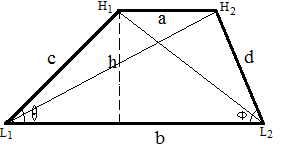# 梯形的面积与周长

## 梯形的面积与周长

2017-01-03 作者: xuzhiping 浏览: 2733 次

### 梯形的性质

1.梯形的上下两底平行

2.梯形的中位线，平行于两底并且等于上下底和的一半

3.等腰梯形对角线相等

### 梯形的判定

1.一组对边平行，另一组对边不平行的四边形是梯形。

2.一组对边平行且不相等的四边形是梯形。

### 梯形的面积和周长

1.梯形面积$$S = h(a+b)/2$$

2.梯形周长$$C = a + b + c + d$$

3.梯形对角线 $$L1H2 = sqrt(h2+(b-sqrt(c2-h2))2)$$

4.梯形对角线 $$H1L2 = sqrt(h2+(b-sqrt(d2-h2))2)$$